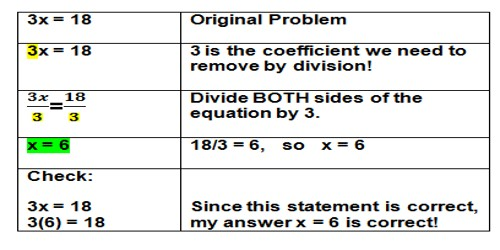Mathematic

# Division EquationsDivision Equations

An equation is a mathematical statement such that the expression on the left side of the equals sign (=) has the same value as the expression on the right side. An example of an equation is 72 ÷ 8 = 9. There are some equations that you can solve in your head quickly. For example – what is the value of y in the equation 2y = 6? Chances are you didn’t need to get out a pencil and paper to calculate that y = 3. You only needed to do one thing to get the answer, divide 6 by 2.

One of the terms in an equation may not be known and needs to be determined. The unknown term may be represented by a letter such as x. (e.g. x ÷ 8 = 9).

The solution of an equation is finding the value of the unknown x. Use the multiplication property of equations to find the value of x. The multiplication property property of equations states that the two sides of an equation remain equal if both sides are multiplied by the same number.

Example:

x ÷ 5 = 2

x ÷ 5 * 5 = 2 * 5

x ÷ 1 = 10

x = 10

Check the answer by substituting the answer (10) back into the equation.

10 ÷ 5 = 2

Solving multi-step equations

1. If necessary, simplify the expressions on each side of the equation, including combining like terms.
2. Get all variable terms on one side and all numbers on the other side using the addition property of equality. (ax + b = c or c = ax + b)
3. Isolate the variable term using the inverse operation or additive inverse (opposite) using the addition property of equality
4. Isolate the variable using the inverse operation or multiplicative inverse (reciprocal) using the multiplication property of equality to write the variable with a coefficient of 1.
5. Check your solution by substituting the value of the variable in the original equation.

Complex, multi-step equations often require multi-step solutions. Before you can begin to isolate a variable, you may need to simplify the equation first. This may mean using the distributive property to remove parentheses, or multiplying both sides of an equation by a common denominator to get rid of fractions. Sometimes it requires both techniques.

Information Source: